# Learn the concept of For Loop in Linux Shell Scripting

0
4019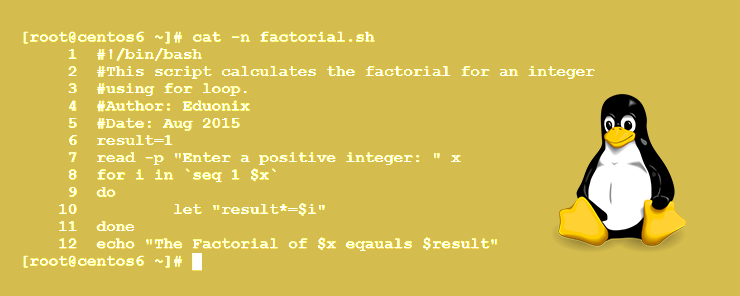There will be cases wherein you need to repeatedly execute a block of code. Such repeated execution of portions of your code is done using what we call Loops. Linux Shell has three types of loops:

• The for loop
• The while loop
• The until loop

Each type of the three has its own syntax and unique usages. So, let’s start with the first type: the for loop.

The for Loop
A very well-known loop type, supported in almost all programming languages.

Syntax:

```for loop_var in list_of_values
do
statement1
statement1
…
statementn
done
```

where:
loop_var is the loop variable that loops (iterates) over all the values in the provided list.
list_of_values is a collection (or range) of all possible values that the loop variable could take.
statements1 through n constitute the loop body; this is the block of code to be executed repeatedly.
do and done are the delimiters that delimit the loop body.

To get the idea closer to your mind, let’s illustrate it by example.

Example
In this example, we are going to write a script that calculates the factorial of an integer using the for loop. From mathematics, we know that the factorial of an integer n is equal to:

n * (n-1) * (n-2) …. * 2 * 1

So, let’s see together how for loop could achieve this. Check the following code: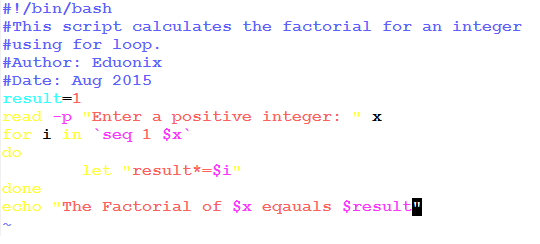Should that work and calculate the factorial of the entered integer value?!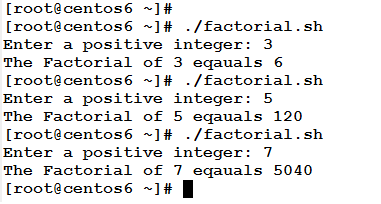Yes, it worked as expected. Now, let’s explain the above code.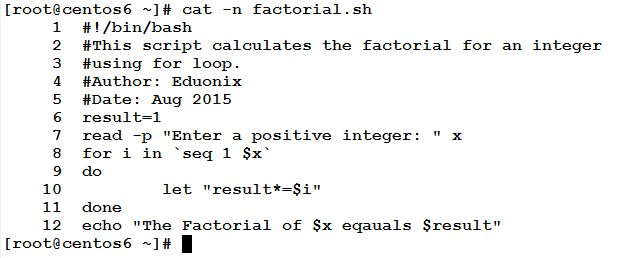• Line 6: initializes the variable result to 1.
• Line 7: uses the read command to prompt the user to enter a positive integer, and assigns the input to the variable x.
• Line 8: starts a for loop with a loop variable i  that iterates over the range of values that comes from the output of expansion of the command seq 1 x
• Lines 9 and 11: are the delimiters of the loop body.
• Line 10: the only statement in the loop body. It uses the let command to make an accumulative multiplication using the *= operator. It multiplies the loop variable i by the variable result, and then stores the result in the variable result.
• Line 12: prints the results back to the user.

* * * * * *

In the previous example, we had a for loop that iterated over a sequence of numeric values. The for loop could also iterate over a list of: filenames, directories, words of a file, process IDs (PIDs). In the next example, we are going to use for loop to rename all the files in a directory.

Example
Given a directory that contains a large number of files. Your manager has asked you to rename all the files in the directory by adding the suffix .backup to the end of each filename.
How would you do this?!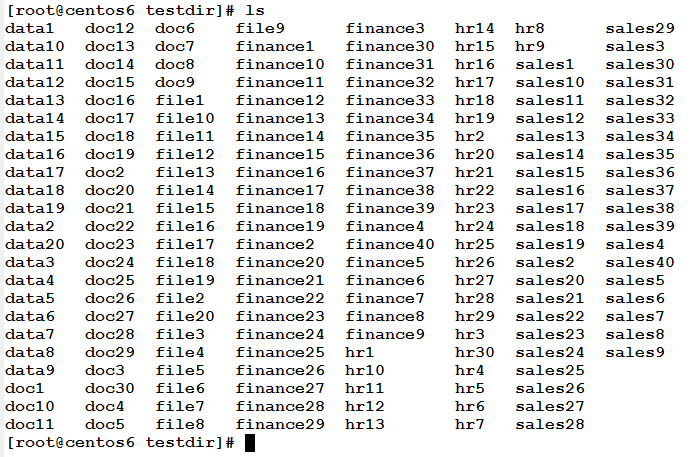You have two choices: the first is to go ahead and start renaming every file, spending a whole day (and 8 cups of coffee), and leaving for home at last at 21:00. The other, is to think as a programmer, and start writing a short script that offloads this burden from your shoulders (and make your manager happy with you finishing this task in 5 minutes). Read the following script.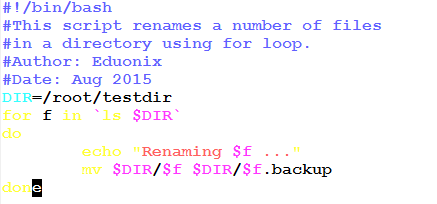Let’s see how this short script will rename all the above files in one execution.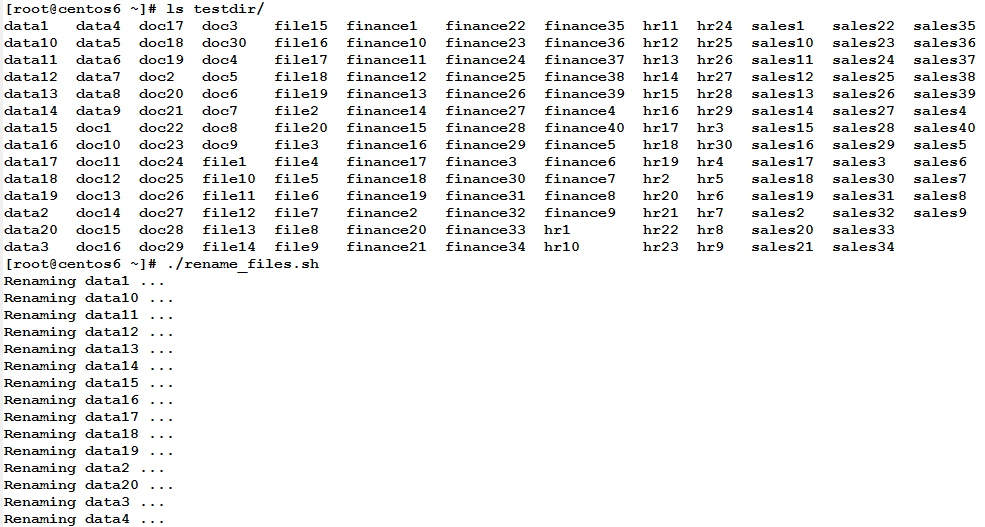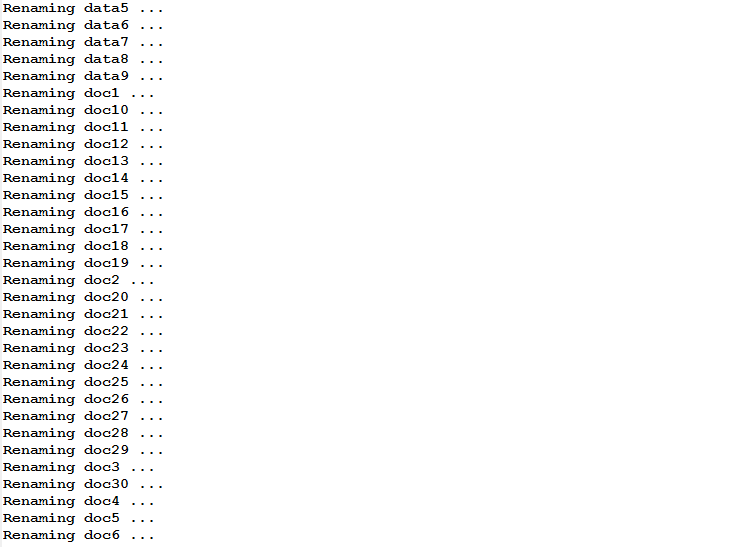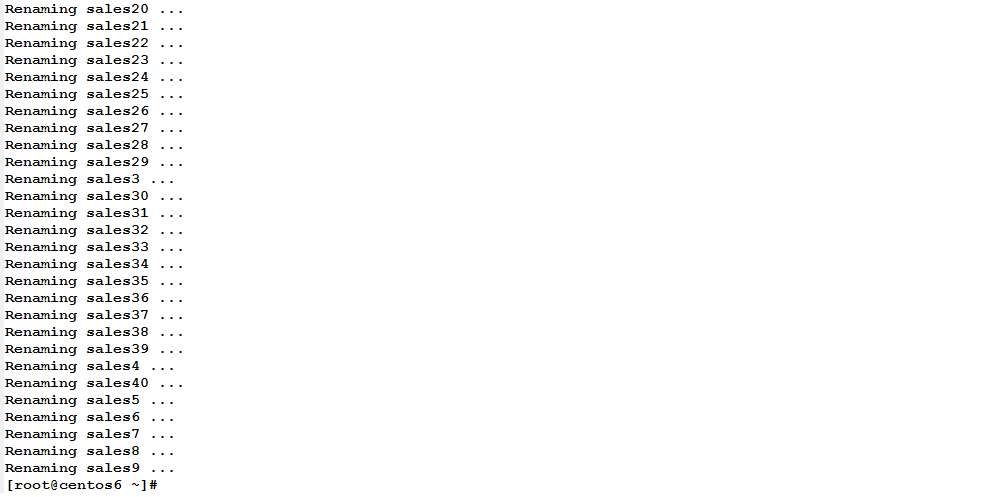If you list the files in this directory again, you should find them renamed.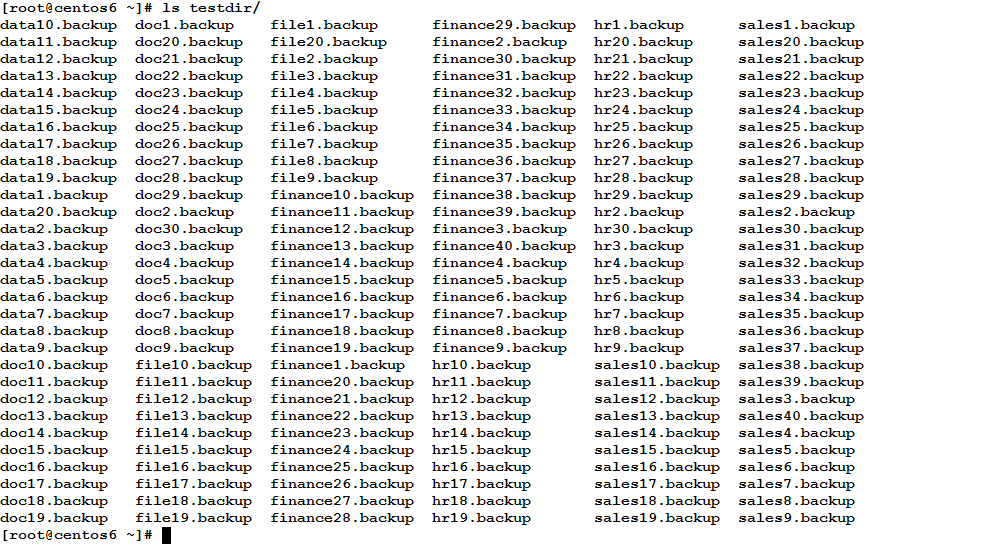Now, to the explanation: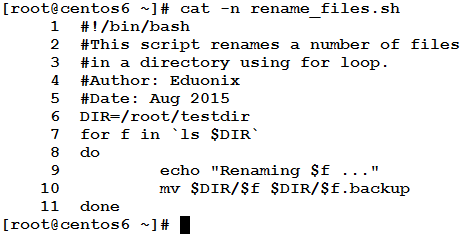• Line 6: defines a variable DIR, and assigns to it the full path of the directory containing the files to be renamed.
• Line 7: starts the for loop. In this loop the loop variable f iterates over the list of files in the directory /root/testdir.
• Lines 8 and 11: the do and done statements that act as loop body delimiters.
• Lines 9 and 10: The loop body:
• Line 9: prints a message that the script is renaming the file.
• Line 10: uses the mv command to rename the file in hand.

So simple and logic, isn’t it?!

* * * * * *

Using for Loop to Kill Specific Type of Processes
Consider a case wherein you need to kill all processes started by a specific user, could for loop do something?! Sure. Let’s consider the following example.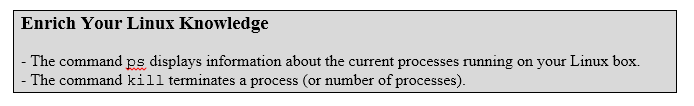Example
Your manager asked you to terminate any process started by the user postgres.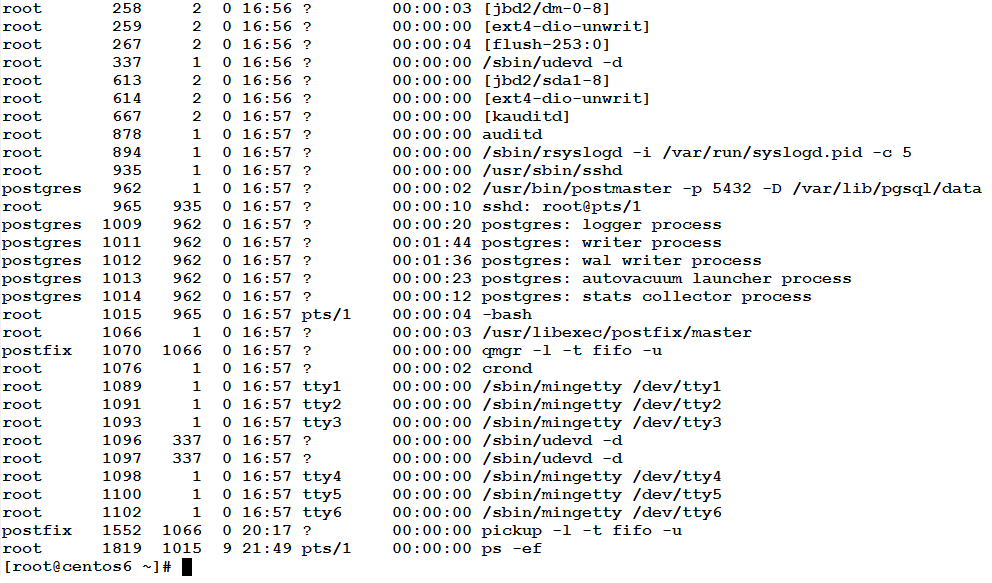Again, you have two choices: either to list such processes using the ps command, and copy each PID and paste manually as an argument to the kill command, or… write the following script.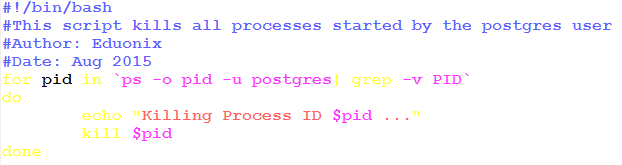Now, let’s see it in action.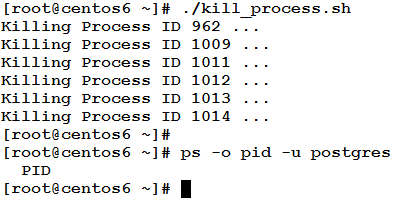All processes have been killed in one script execution. Now, let’s explain things:• Line 5: defines the for loop whose variable pid iterates on the output from the command:
`ps –o pid –u postgres | grep –v PID`

which prints the PIDs (process IDs) of the processes owned by the user postgres.

• Lines 6 and 9: the do and done statements that mark the start and end of the loop body.
• Lines 7 and 8: the loop body to be executed repeatedly for each PID.
• Line 7: prints a message that the Process with the given PID will be killed.
• Line 8: uses the kill command to terminate the process.

Isn’t it so beautiful?!

* * * * * *

In this article, we have started talking about Loops. There are 3 types of loops supported in Linux Shells. Of the three types, we have talked about the for loop. The for loop is very useful when we need to iterate over a range or a list of values. We have seen some situations wherein the for loop did a great job and saved time and effort. In the next article, we are going to talk on another great type: the while loop. So, see you there!!